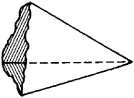# Trihedral Angle

## trihedral angle

[trī¦hē·drəl ′aŋ·gəl]
(mathematics)
A polyhedral angle with three faces.
McGraw-Hill Dictionary of Scientific & Technical Terms, 6E, Copyright © 2003 by The McGraw-Hill Companies, Inc.
The following article is from The Great Soviet Encyclopedia (1979). It might be outdated or ideologically biased.

## Trihedral Angle

a part of space bounded by a triangular pyramid of infinite altitude (see Figure 1). In English the term “trihedral angle” is often applied to such a triangular pyramid, rather than the space it bounds.Figure 1

The faces of the pyramid are called the faces of the trihedral angle, and the vertex of the pyramid is called the vertex of the trihedral angle. The rays along which the faces intersect are known as the edges of the trihedral angle. The edges form the plane angles of the trihedral angle, and the faces form the dihedral angles of the trihedral angle. We usually consider trihedral angles whose dihedral angles are less than π. Every plane angle of such a trihedral angle is less than the sum of the other two plane angles and greater than their difference.